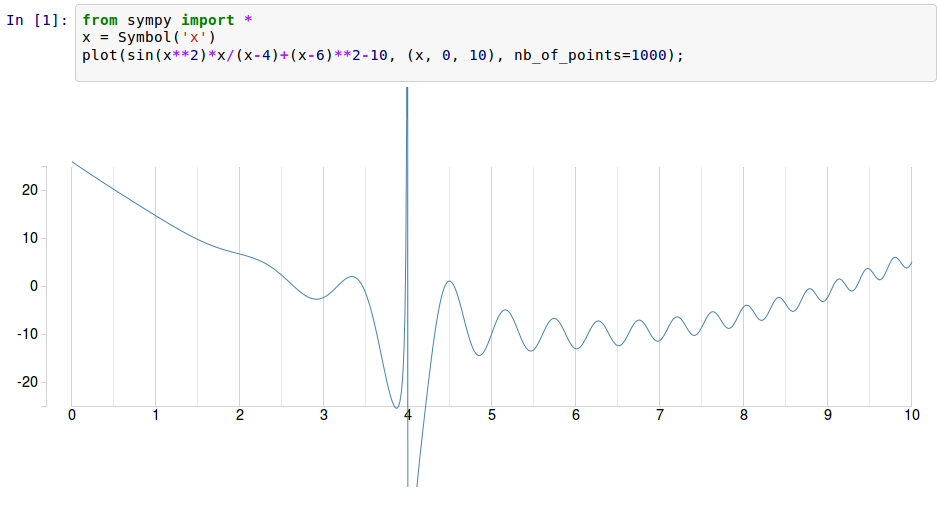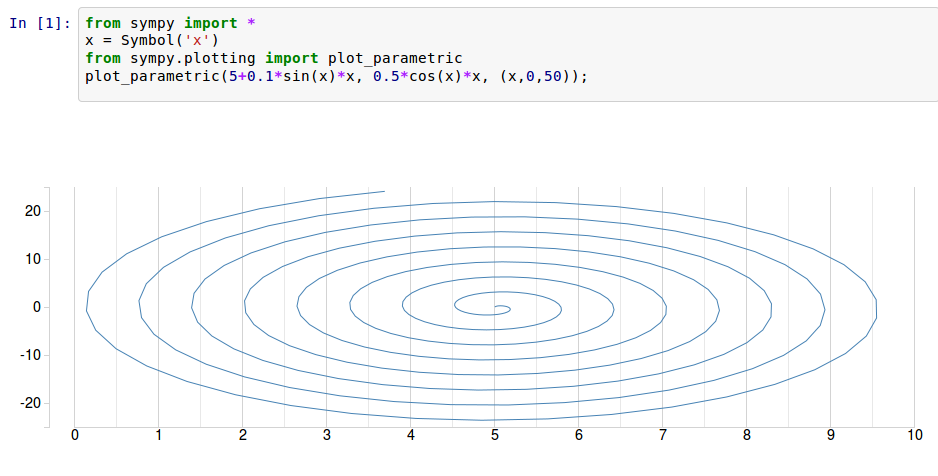About a year ago I contributed a plotting module to SymPy, which was extended with some nice features thanks to a GSoC project just after that. The module supports multiple backends, but for the moment only `matplotlib` is implemented. It would have been simpler if we had decided to support only `matplotlib`, but that would mean limiting ourselves to producing math graphics with a tool designed for engineers. Moreover, `matplotlib` does not support all modes of presentation that we would like to have (e.g. nice renderings of surfaces for when we are solving systems of nonlinear equations). Finally, given its `Matlab` heritage and dedication to backward compatibility is rather unattractive in its default settings.

Hence I started playing around with `D3.js` in IPython. I was told that the IPython team will work on a canonical way to represent objects through visualization libraries after the release of version 1.0, but that does not mean that we can not concort together some “Not The Right Way” solution now. Moreover it would permit me to show how easy it is to add a new backend to the SymPy plotting module.

Anyway, if you want to obtain this:it would be sufficient to make a new backend that uses `publish_html` and `publish_javascript` in its `show()` method.

Start with

```class IPythonD3Backend(BaseBackend):
def __init__(self, parent):
super(IPythonD3Backend, self).__init__(parent)
are_3D = [s.is_3D for s in self.parent._series]
if any(are_3D):
raise ValueError('The D3 backend can not do 3D.')
```

For the moment we will restrict ourselves to single lines, as this is just a prototype:

```    def process_series(self):
parent = self.parent

for s in self.parent._series:
# Create the collections
if s.is_2Dline:
self.data = "[%s]"%(", ".join("[%s, %s]"%_ for _ in zip(*s.get_points())))
else:
raise ValueError('The D3 backend does not support this type of plot.')
```

and in `show` we construct the html and javascript code that we want to publish.

```    def show(self):
global _ipython_div_counter
from IPython.core.display import publish_javascript, publish_html
self.process_series()
publish_html("""
<style>
/* tell the SVG path to be a thin blue line without any area fill */
path {
stroke: steelblue;
stroke-width: 1;
fill: none;
}

.axis {
shape-rendering: crispEdges;
}

.y.axis .minor, .x.axis .minor {
stroke-opacity: .5;
}

.x.axis line, .x.axis path, .y.axis line, .y.axis path {
stroke: lightgrey;
}
</style>

<div id="sympy_d3_plot_%d" class="aGraph" style="position:absolute;top:0px;left:0; float:left;"></div
"""%_ipython_div_counter)
publish_javascript("""
var script = document.createElement("script");
script.src = "http://d3js.org/d3.v3.min.js";
document.body.appendChild(script);

var m = [80, 80, 80, 80]; // margins
var w = 1000 - m - m; // width
var h = 400 - m - m; // height

var data = %s;

var x = d3.scale.linear().domain([0, 10]).range([0, w]);
var y = d3.scale.linear().domain([-25, 25]).range([h, 0]);

var line = d3.svg.line()
.x(function(d) {
return x(d);
})
.y(function(d) {
return y(d);
})

var graph = d3.select("#sympy_d3_plot_%d").append("svg:svg")
.attr("width", w + m + m)
.attr("height", h + m + m)
.append("svg:g")
.attr("transform", "translate(" + m + "," + m + ")");

var xAxis = d3.svg.axis().scale(x).tickSize(-h).tickSubdivide(true);
graph.append("svg:g")
.attr("class", "x axis")
.attr("transform", "translate(0," + h + ")")
.call(xAxis);

var yAxisLeft = d3.svg.axis().scale(y).ticks(4).orient("left");
graph.append("svg:g")
.attr("class", "y axis")
.attr("transform", "translate(-25,0)")
.call(yAxisLeft);

graph.append("svg:path").attr("d", line(data));
""" % (self.data, _ipython_div_counter))
_ipython_div_counter += 1

def save(self, path):
pass

def close(self):
pass
```

The only slightly nontrivial part is loading the D3 library, which is done with the following snippet:

```        var script = document.createElement("script");
script.src = "http://d3js.org/d3.v3.min.js";
document.body.appendChild(script);
```

And the nice thing is that this works for all possible line charts (so parametric charts do not need additional treatment):Obviously much more can be done, but I will probably wait for IPython 1.0 before writing this new backend.